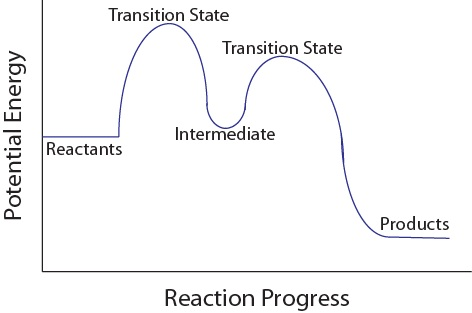Chapter 17. Kinetics

# Reaction Mechanisms

Jessie A. Key

### Learning Objectives

• To gain an understanding of reaction mechanisms, including the concepts of elementary steps and molecularity.
• To become familiar with reaction potential energy diagrams.
• To gain an understanding of rate-determining steps in multistep reactions.

For us to truly understand a chemical reaction, including its rate, it would be ideal to see the exact bond-making and bond-breaking steps that occur at the molecular level – the reaction mechanism. Unfortunately, we cannot watch reactions occurring at the molecular level, but we can infer these events using kinetics and other chemical methods.

## Elementary Steps

Each event that occurs in a chemical reaction as a result of an effective collision is known as an elementary step. The total number of molecules that participate in the effective collision of an elementary step is known as its molecularity. Molecularity can be used to classify elementary steps into three categories:

Unimolecular – Only one molecule participates

Bimolecular – Two molecules participate

Termolecular – Three molecules participate

There are only three main categories of molecularity because it is rare that more than three molecules participate simultaneously in an effective collision.

## Elementary Steps and Rate Laws

A complete chemical reaction may occur in one or more elementary steps, each having its own rate law. The rate of a single elementary step can be derived directly from its stoichiometric equation, since it is an individual effective collision describing a single bond-breaking or bond-forming event. This explains why the rate law of an overall reaction, potentially involving several steps, does not necessarily correlate to the stoichiometry of its balanced chemical equation. For example:

5 Br(aq) + BrO3(aq) + 6 H+(aq) → 3 Br2(ℓ) + 3 H2O(ℓ)

Rate = k[Br][BrO3][H+]2

However, the rate law for an elementary step is determined from its molecularity: the number of molecules involved in the single effective collision (Table 17.2 “Elementary Steps and Their Rate Laws”). For the following elementary step:

A → B

Rate = k[A]

This is a unimolecular step, and as the concentration of reactant A molecules increases, the number of effective collisions also increases.

Table 17.2 Elementary Steps and Their Rate Laws

 Elementary Step Molecularity Rate Law A → B Unimolecular Rate = k[A] 2 A → C Bimolecular Rate = k[A]2 2 A + D → E Termolecular Rate = k[A]2[D]

## Multistep Mechanisms

The overall reaction process often corresponds to a series of two or more elementary steps, which must always add up to give the overall balanced chemical equation. For example, in the following elimination reaction (a type of reaction typically discussed in introductory organic chemistry courses):

C4H9Br + H2O → C4H8 + H3O+ + Br

This reaction process proceeds in two elementary steps and would therefore be called a two-step mechanism. In the first step, bromide leaves from the starting material to give the cation C4H9+. In the second step, C4H9+ reacts with water to generate the product C4H8 and H3O+.

1. C4H9Br ⇌ C4H9+ + Br                   (slow)

2. C4H9+ + H2O ⇌ C4H8 + H3O+

Net: C4H9Br + H2O → C4H8 + H3O+ + Br

The cation C4H9+ is called an intermediate, since it does not appear in the overall balanced equation and is generated in one elementary step but used up in a subsequent step. A potential energy diagram for this multistep reaction can be drawn as shown in Figure 17.12 “Multistep Reaction Potential Energy Diagram.” Notice each step has its own activation energy, and transition state (or activated complex), which is the highest-energy transitional point in the elementary step. Transition states are very unstable (high energy) as bonds are in the process of breaking or forming, and therefore transition states cannot be isolated. Intermediates are more stable than transition states and can sometimes be isolated and characterized by certain techniques.

Figure 17.12. Multistep Reaction Potential Energy DiagramMultistep reaction potential energy diagram showing the intermediate

## The Rate-Determining Step

For multistep mechanisms, there is often one step that is significantly slower than the other steps. This slowest step is referred to as the rate-determining step, as it limits the rate of the entire reaction. An analogy that illustrates this concept is an hourglass having two different sized openings. The rate of the sand falling to the bottom-most chamber is determined by the smaller of the two openings (Figure 17.13 “Rate-Determining Point in an Hourglass”). Similarly, the rate law of the overall reaction is determined from its rate-determining slowest step.

Figure 17.13.  Rate-Determining Point in an Hourglass

Double hourglass with one opening smaller than the other, which determines rate

Example 9

The following reaction occurs in a two-step mechanism:

A → B + C (slow)

A + C → B + D (fast)

1. Determine the overall reaction equation.

2. Write the rate law for the overall reaction.

Solution

1. Add the two elementary steps together and cancel out any intermediates to give the overall reaction: 2 A → 2 B + D

2. Rate = k[A]2

### Key Takeaways

• The overall reaction process often corresponds to a series of more than one elementary step, which must always add up to give the overall balanced chemical equation.
• Each step has its own activation energy and transition state.
• The slowest step of a multistep reaction is the rate-determining step.

## LicenseReaction Mechanisms by Jessie A. Key is licensed under a Creative Commons Attribution 4.0 International License, except where otherwise noted.